# Chapter 5 ARRAYS Why Do We Need Arrays

• Slides: 42Chapter 5: ARRAYS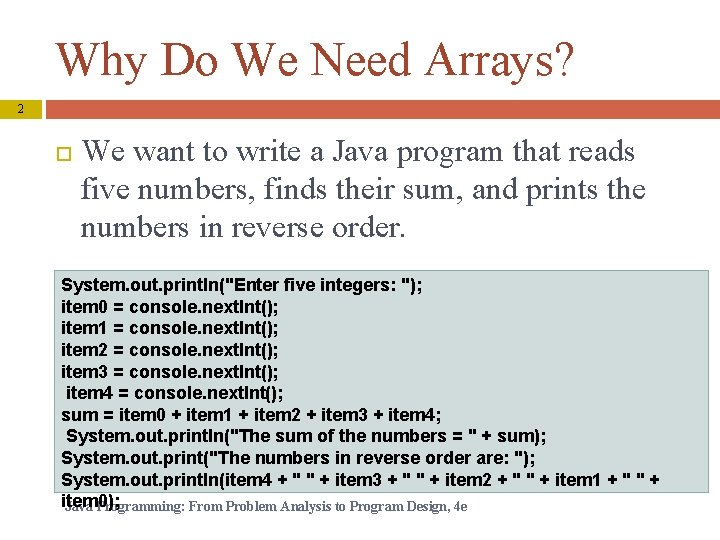Why Do We Need Arrays? 2 We want to write a Java program that reads five numbers, finds their sum, and prints the numbers in reverse order. System. out. println("Enter five integers: "); item 0 = console. next. Int(); item 1 = console. next. Int(); item 2 = console. next. Int(); item 3 = console. next. Int(); item 4 = console. next. Int(); sum = item 0 + item 1 + item 2 + item 3 + item 4; System. out. println("The sum of the numbers = " + sum); System. out. print("The numbers in reverse order are: "); System. out. println(item 4 + " " + item 3 + " " + item 2 + " " + item 1 + " " + item 0); Java Programming: From Problem Analysis to Program Design, 4 e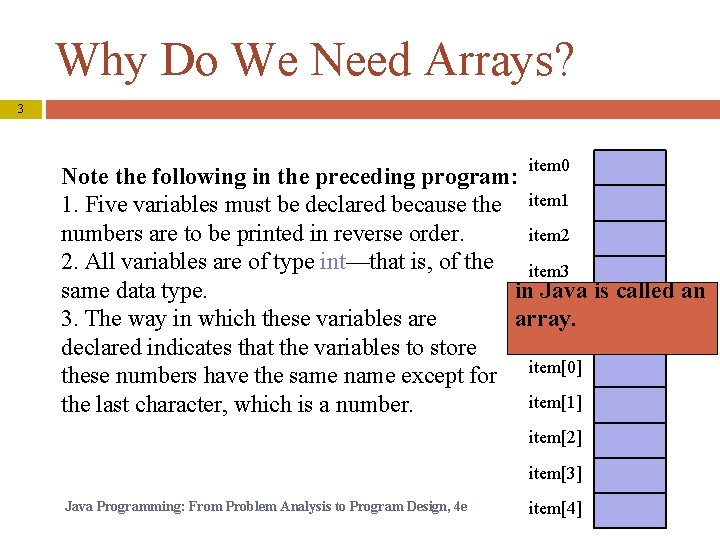Why Do We Need Arrays? 3 item 0 Note the following in the preceding program: 1. Five variables must be declared because the item 1 numbers are to be printed in reverse order. item 2 2. All variables are of type int—that is, of the item 3 in Java is called an same data type. item 4 array. 3. The way in which these variables are declared indicates that the variables to store these numbers have the same name except for item item the last character, which is a number. item item Java Programming: From Problem Analysis to Program Design, 4 e item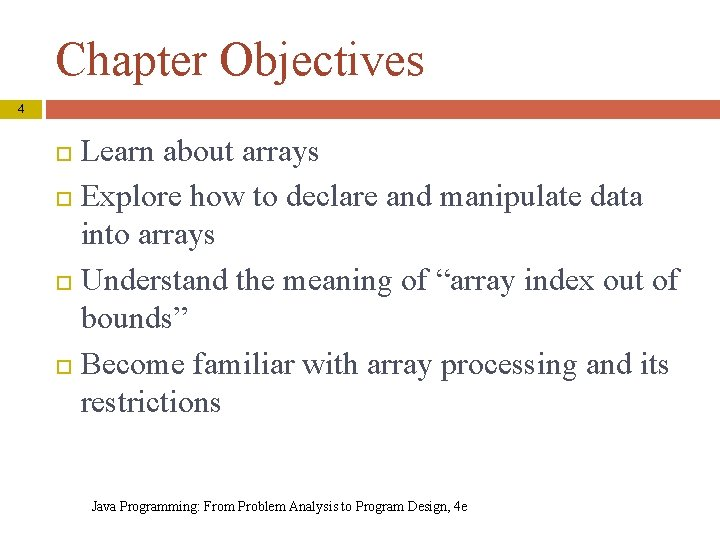Chapter Objectives 4 Learn about arrays Explore how to declare and manipulate data into arrays Understand the meaning of “array index out of bounds” Become familiar with array processing and its restrictions Java Programming: From Problem Analysis to Program Design, 4 eArray 5 Definition: structured data type with a fixed number of elements Elements of an array are also called components of the array Every element is of the same type Elements are accessed using their relative positions in the array Java Programming: From Problem Analysis to Program Design, 4 eOne-Dimensional Arrays 6 Java Programming: From Problem Analysis to Program Design, 4 e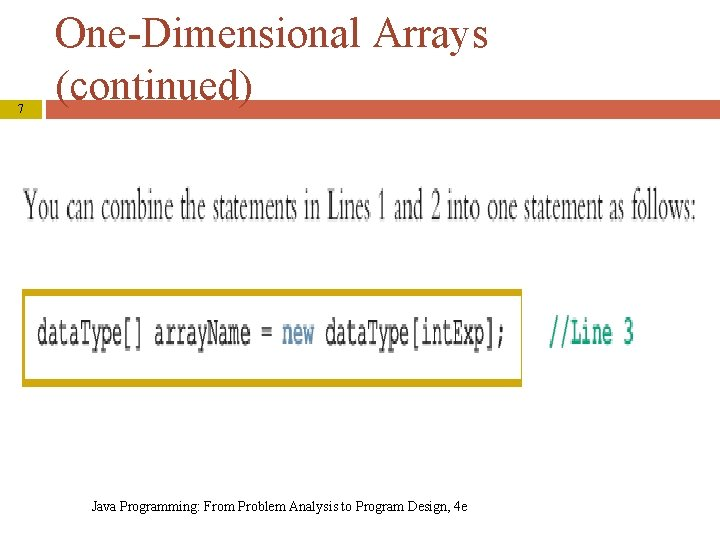7 One-Dimensional Arrays (continued) Java Programming: From Problem Analysis to Program Design, 4 eArrays 8 • Array num: int[] num = new int; When an array is instantiated, Java automatically initializes its elements to their default values. numeric arrays are initialized to 0, char arrays are initialized to the null character, which is 'u 0000', boolean arrays are initialized to false. Java Programming: From Problem Analysis to Program Design, 4 e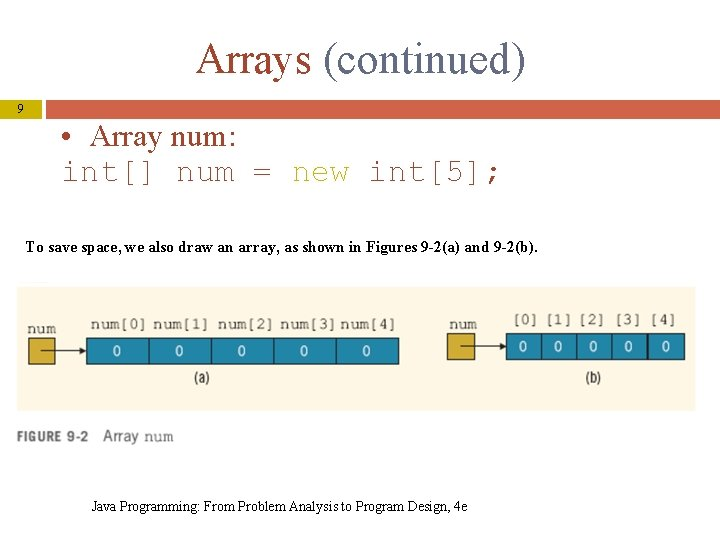Arrays (continued) 9 • Array num: int[] num = new int; To save space, we also draw an array, as shown in Figures 9 -2(a) and 9 -2(b). Java Programming: From Problem Analysis to Program Design, 4 e10 One-Dimensional Arrays (continued) int. Exp = number of components in array >= 0 0 <= index. Exp < int. Exp Java Programming: From Problem Analysis to Program Design, 4 eArray List 11 Java Programming: From Problem Analysis to Program Design, 4 eArray List (continued) 12 Java Programming: From Problem Analysis to Program Design, 4 e13 Array List (continued) Java Programming: From Problem Analysis to Program Design, 4 e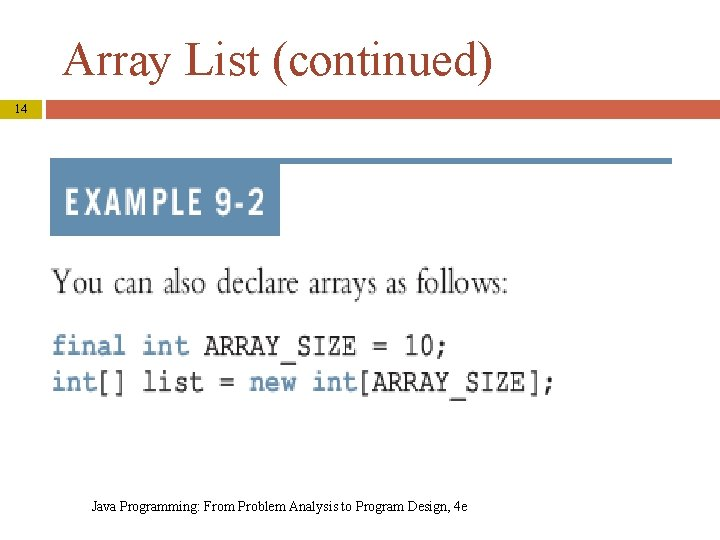Array List (continued) 14 Java Programming: From Problem Analysis to Program Design, 4 e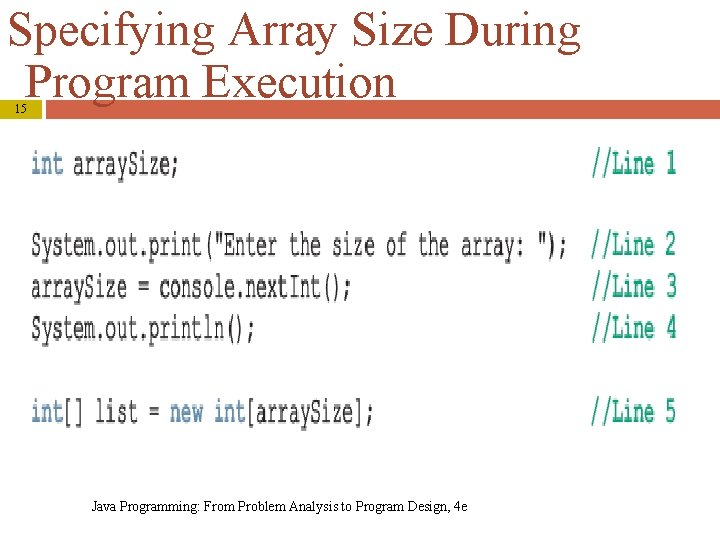Specifying Array Size During Program Execution 15 Java Programming: From Problem Analysis to Program Design, 4 e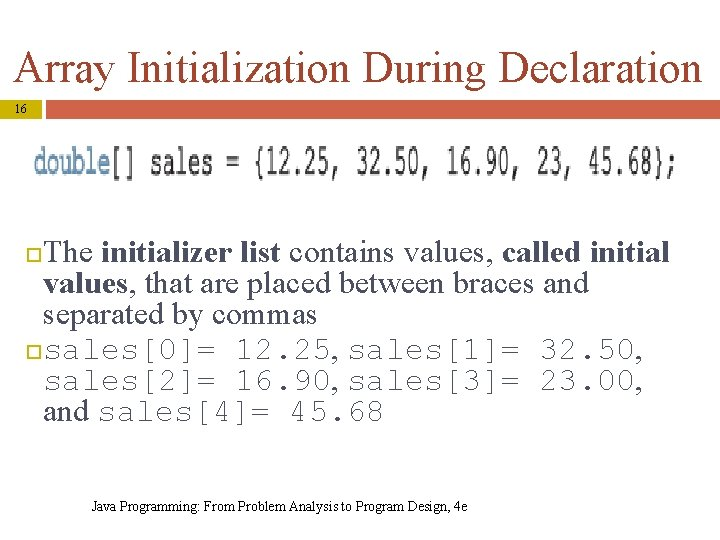Array Initialization During Declaration 16 The initializer list contains values, called initial values, that are placed between braces and separated by commas sales= 12. 25, sales= 32. 50, sales= 16. 90, sales= 23. 00, and sales= 45. 68 Java Programming: From Problem Analysis to Program Design, 4 eArray Initialization During Declaration (continued) 17 int[] list = {10, 20, 30, 40, 50, 60}; When declaring and initializing arrays, the size of the array is determined by the number of initial values within the braces. If an array is declared and initialized simultaneously, we don’t use the operator new to instantiate the array object Java Programming: From Problem Analysis to Program Design, 4 eArrays and the Instance Variable length 18 Associated with each array that has been instantiated, there is a public (final) instance variable length The variable length contains the size of the array The variable length can be directly accessed in a program using the array name and the dot operator Java Programming: From Problem Analysis to Program Design, 4 e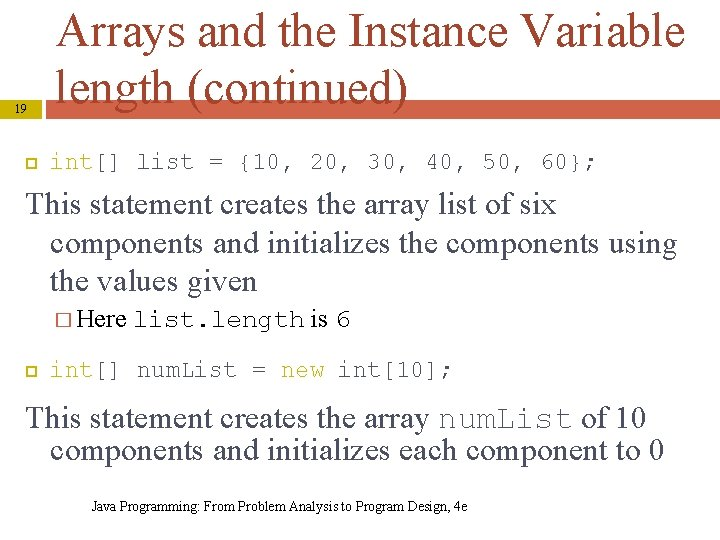19 Arrays and the Instance Variable length (continued) int[] list = {10, 20, 30, 40, 50, 60}; This statement creates the array list of six components and initializes the components using the values given � Here list. length is 6 int[] num. List = new int; This statement creates the array num. List of 10 components and initializes each component to 0 Java Programming: From Problem Analysis to Program Design, 4 e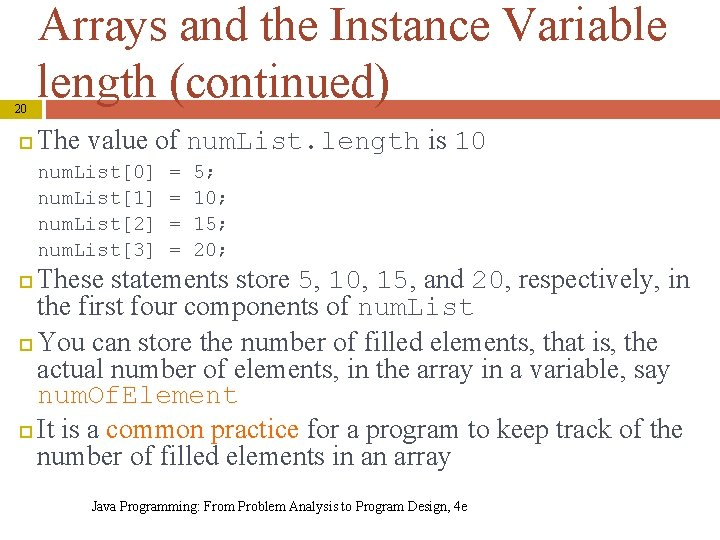20 Arrays and the Instance Variable length (continued) The value of num. List. length is 10 num. List num. List num. List num. List = = 5; 10; 15; 20; These statements store 5, 10, 15, and 20, respectively, in the first four components of num. List You can store the number of filled elements, that is, the actual number of elements, in the array in a variable, say num. Of. Element It is a common practice for a program to keep track of the number of filled elements in an array Java Programming: From Problem Analysis to Program Design, 4 e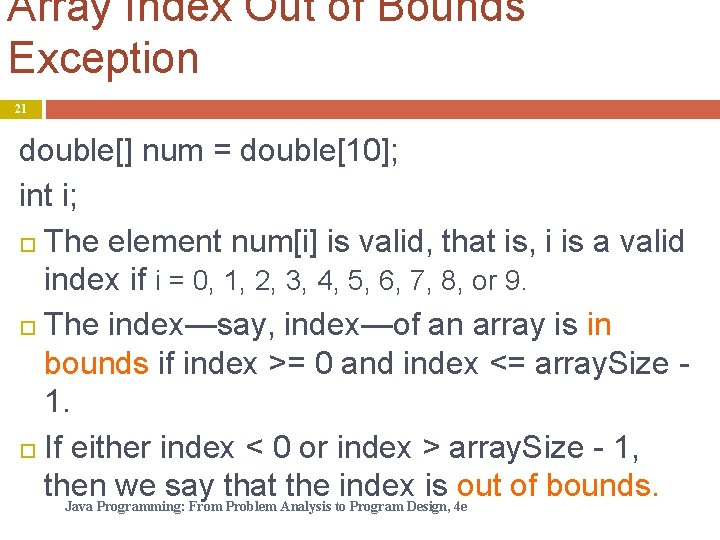Array Index Out of Bounds Exception 21 double[] num = double; int i; The element num[i] is valid, that is, i is a valid index if i = 0, 1, 2, 3, 4, 5, 6, 7, 8, or 9. The index—say, index—of an array is in bounds if index >= 0 and index <= array. Size 1. If either index < 0 or index > array. Size - 1, then we say that the index is out of bounds. Java Programming: From Problem Analysis to Program Design, 4 e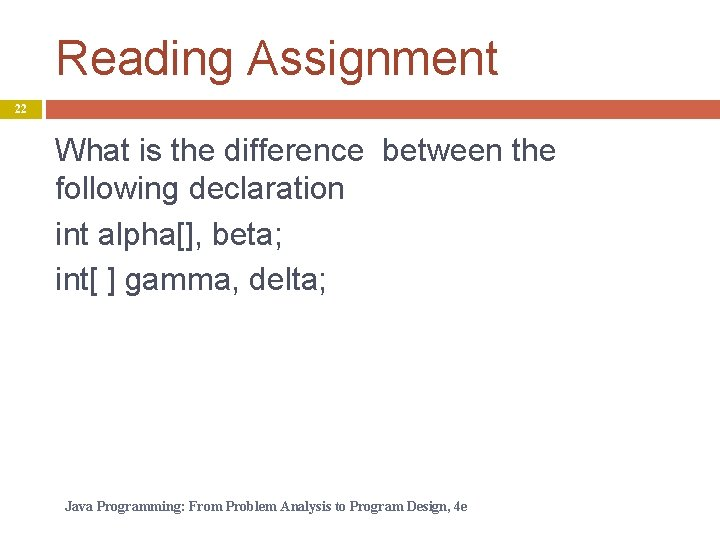Reading Assignment 22 What is the difference between the following declaration int alpha[], beta; int[ ] gamma, delta; Java Programming: From Problem Analysis to Program Design, 4 e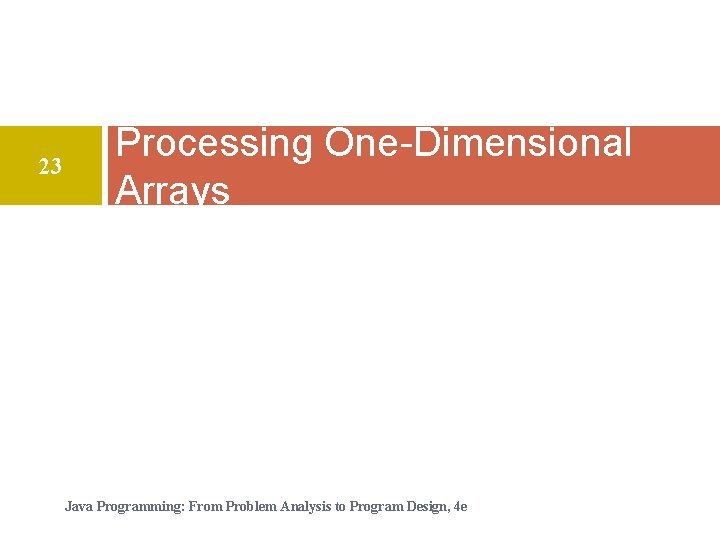23 Processing One-Dimensional Arrays Java Programming: From Problem Analysis to Program Design, 4 e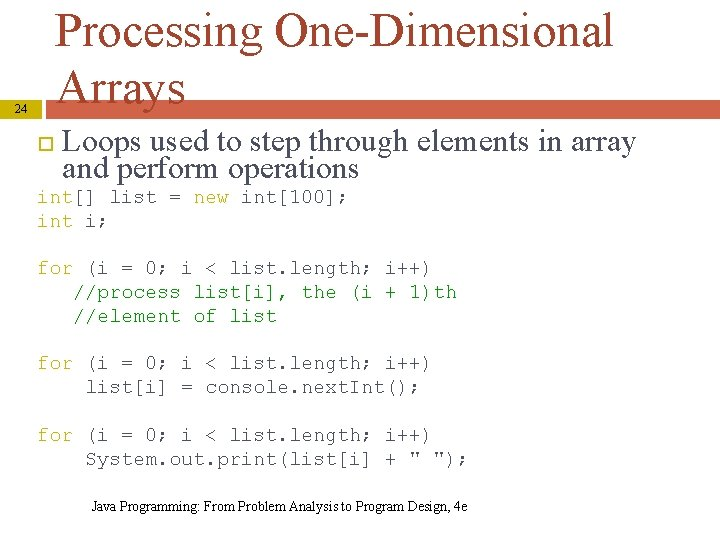Processing One-Dimensional Arrays 24 Loops used to step through elements in array and perform operations int[] list = new int; int i; for (i = 0; i < list. length; i++) //process list[i], the (i + 1)th //element of list for (i = 0; i < list. length; i++) list[i] = console. next. Int(); for (i = 0; i < list. length; i++) System. out. print(list[i] + " "); Java Programming: From Problem Analysis to Program Design, 4 e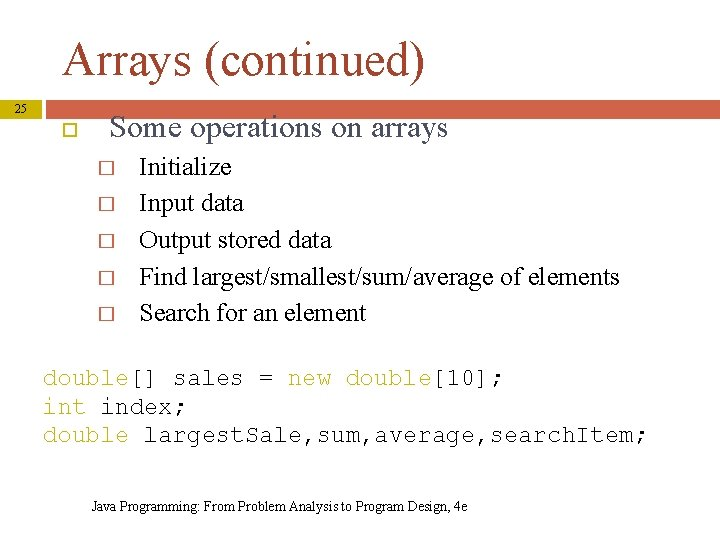Arrays (continued) 25 Some operations on arrays � � � Initialize Input data Output stored data Find largest/smallest/sum/average of elements Search for an element double[] sales = new double; int index; double largest. Sale, sum, average, search. Item; Java Programming: From Problem Analysis to Program Design, 4 e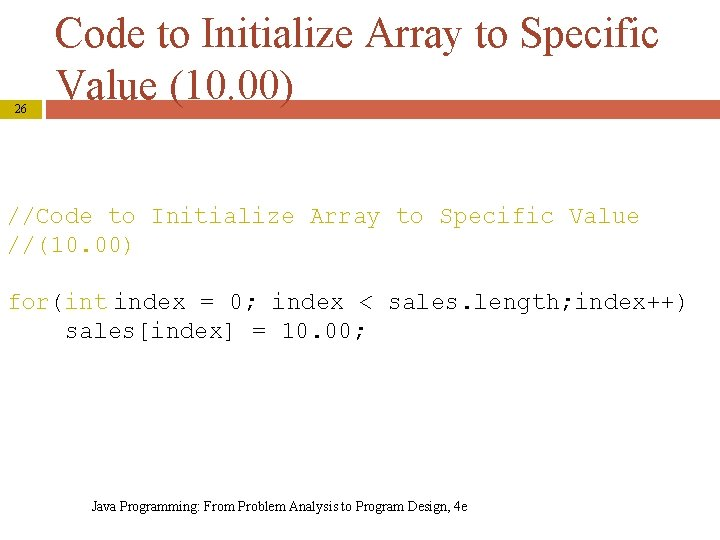26 Code to Initialize Array to Specific Value (10. 00) //Code to Initialize Array to Specific Value //(10. 00) for(int index = 0; index < sales. length; index++) sales[index] = 10. 00; Java Programming: From Problem Analysis to Program Design, 4 e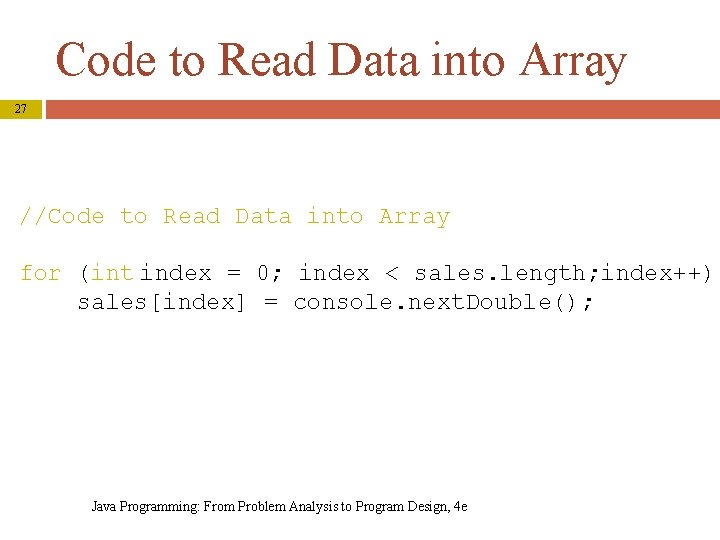Code to Read Data into Array 27 //Code to Read Data into Array for (int index = 0; index < sales. length; index++) sales[index] = console. next. Double(); Java Programming: From Problem Analysis to Program Design, 4 e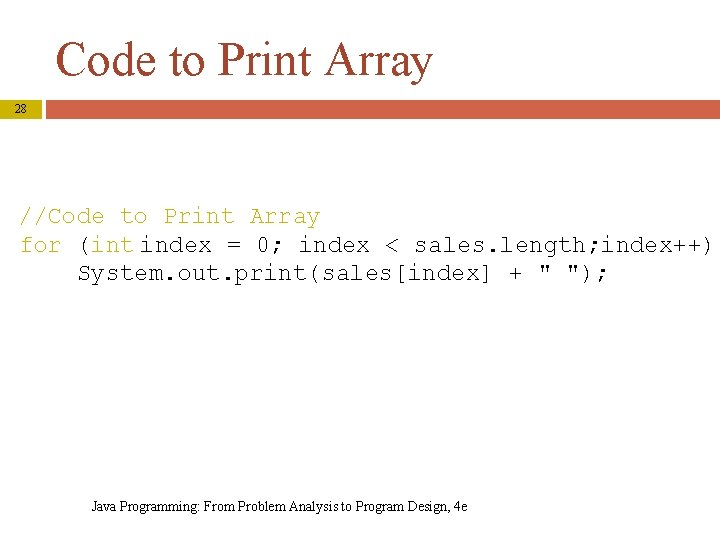Code to Print Array 28 //Code to Print Array for (int index = 0; index < sales. length; index++) System. out. print(sales[index] + " "); Java Programming: From Problem Analysis to Program Design, 4 e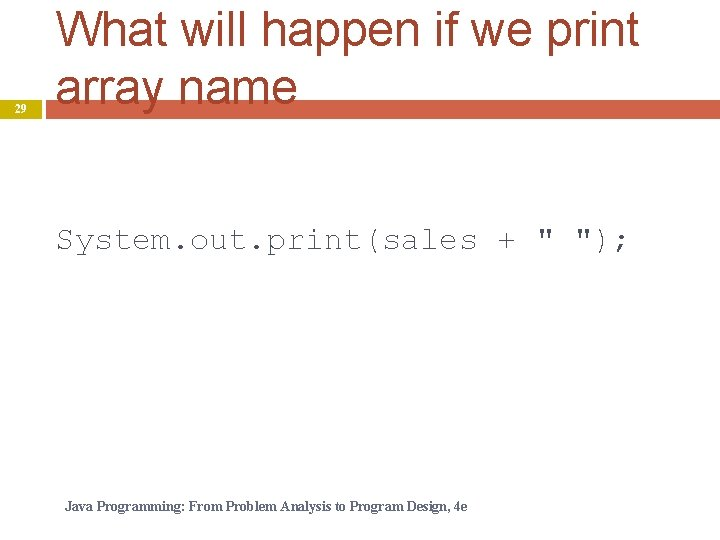29 What will happen if we print array name System. out. print(sales + " "); Java Programming: From Problem Analysis to Program Design, 4 e30 Code to Find Sum and Average of Array sum = 0; for (int index = 0; index < sales. length; index++) sum = sum + sales[index]; if (sales. length != 0) average = sum / sales. length; else average = 0. 0; Java Programming: From Problem Analysis to Program Design, 4 e31 Determining Largest Element in Array max. Index = 0; for (int index = 1; index < sales. length; index++) if (sales[max. Index] < sales[index]) max. Index = index; largest. Sale = sales[max. Index]; Java Programming: From Problem Analysis to Program Design, 4 eDetermining Largest Element in Array (continued) 32 Java Programming: From Problem Analysis to Program Design, 4 eDetermining Largest Element in Array (continued) 33 Java Programming: From Problem Analysis to Program Design, 4 eSearching for specific 34 Search for 10 Search starts at the first element in the list, that is, at list This time, the search item, which is 10, is compared with every item in the list; eventually, no more data is left in the list to compare with the search item; this is an unsuccessful search Java Programming: From Problem Analysis to Program Design, 4 e 3435 search. Item = 10; //can be parameter to a method int list. Length = sales. length; int loc; boolean found = false; loc = 0; while (loc < list. Length && !found) if (sales[loc] == search. Item) found = true; else loc++; if (found) System. out. print(loc); else System. out. print(“not found”); Java Programming: From Problem Analysis to Program Design, 4 e 35Array Index Out of Bounds 36 Array in bounds if: 0 <= index <= array. Size – 1 If index < 0 or index > array. Size: Array. Index. Out. Of. Bounds. Exception exception is thrown Base address: memory location of first component in array Java Programming: From Problem Analysis to Program Design, 4 e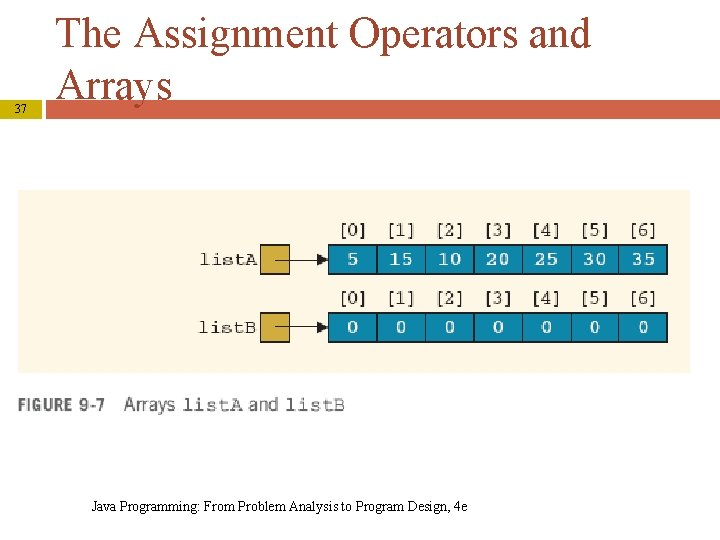37 The Assignment Operators and Arrays Java Programming: From Problem Analysis to Program Design, 4 e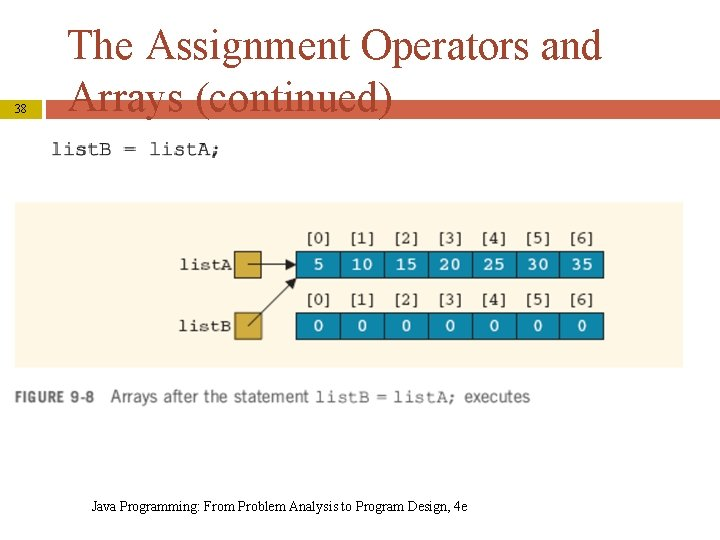38 The Assignment Operators and Arrays (continued) Java Programming: From Problem Analysis to Program Design, 4 e39 The Assignment Operators and Arrays (continued) Java Programming: From Problem Analysis to Program Design, 4 e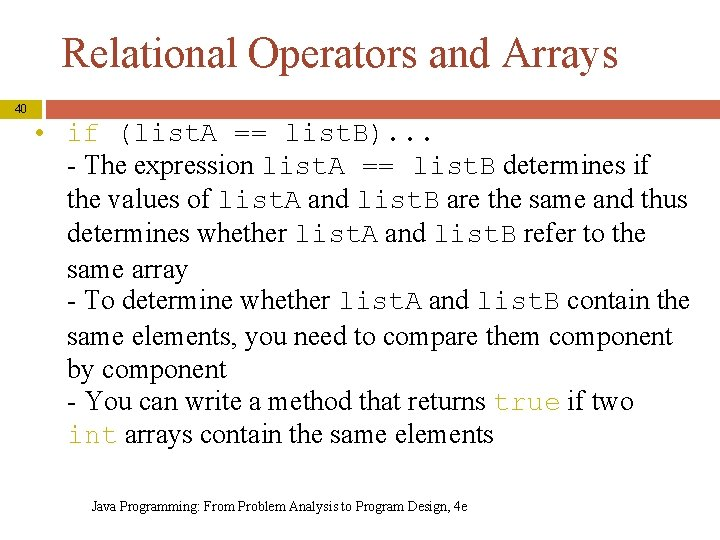Relational Operators and Arrays 40 • if (list. A == list. B). . . - The expression list. A == list. B determines if the values of list. A and list. B are the same and thus determines whether list. A and list. B refer to the same array - To determine whether list. A and list. B contain the same elements, you need to compare them component by component - You can write a method that returns true if two int arrays contain the same elements Java Programming: From Problem Analysis to Program Design, 4 e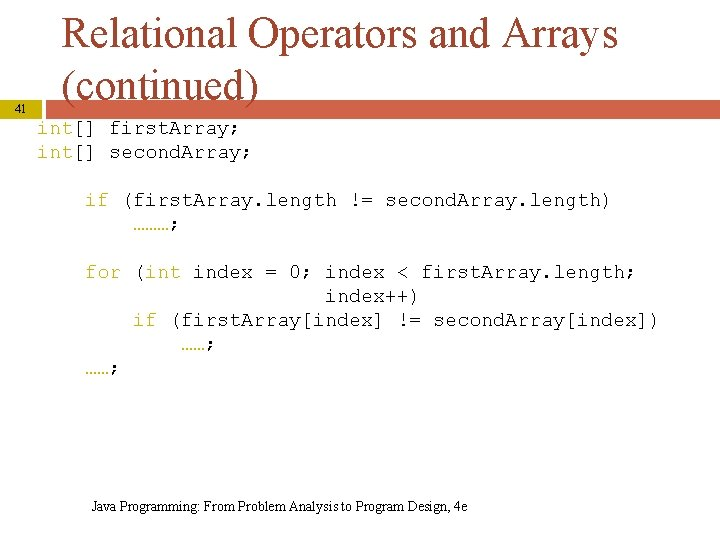41 Relational Operators and Arrays (continued) int[] first. Array; int[] second. Array; if (first. Array. length != second. Array. length) ………; for (int index = 0; index < first. Array. length; index++) if (first. Array[index] != second. Array[index]) ……; Java Programming: From Problem Analysis to Program Design, 4 e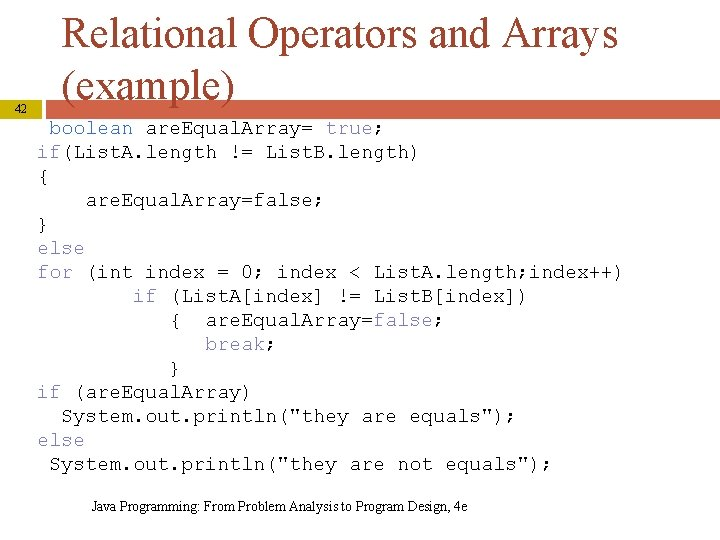42 Relational Operators and Arrays (example) boolean are. Equal. Array= true; if(List. A. length != List. B. length) { are. Equal. Array=false; } else for (int index = 0; index < List. A. length; index++) if (List. A[index] != List. B[index]) { are. Equal. Array=false; break; } if (are. Equal. Array) System. out. println("they are equals"); else System. out. println("they are not equals"); Java Programming: From Problem Analysis to Program Design, 4 e